• 数据结构之查找哈希查找哈希函数构造方法冲突解决办法哈希查找分析 哈希查找 哈希查找是通过设定的哈希函数H(key)和处理冲突的办法将关键字映射的一个地址集（区间），并将关键字在地址集中的“像”作为在表中的存储...

哈希查找

哈希查找是通过设定的哈希函数H(key)和处理冲突的办法将关键字映射的一个地址集（区间），并将关键字在地址集中的“像”作为在表中的存储地址，这个表就是哈希表，对应的这个影响过程就是哈希造表或者散列。

哈希函数构造方法

• 直接定址法
H(key)=a*key+b
• 除数求余法
H(key)=key mod p;(p<m)
• 数字分析法
观察关键字的数字分布，取其中几位作为哈希地址。
• 随机数法
H(key)=randm(key)
• 平方取中法
• 折叠法

冲突解决办法

• 开放定址法：
Hi=（H(key)+di）mod m；
m为哈希表长。
其中di为增量序列有下面两类探测方法：
• 再链接法：

将所有经过哈希函数映射后的相同地址存储在同一线性表中。

哈希查找分析

展开全文数据结构 哈希表
• 哈希查找1. DS哈希查找--链地址法题目描述输入输出输入样例输出样例提示参考代码2. DS哈希查找—线性探测再散列题目描述输入输出输入样例输出样例参考代码3. DS哈希查找—二次探测再散列题目描述输入输出输入样例...

1. DS哈希查找–链地址法

题目描述

给出一个数据序列，建立哈希表，采用求余法作为哈希函数，模数为11，哈希冲突用链地址法和表头插入

如果首次查找失败，就把数据插入到相应的位置中

实现哈希查找功能

输入

第一行输入n，表示有n个数据
第二行输入n个数据，都是自然数且互不相同，数据之间用空格隔开
第三行输入t，表示要查找t个数据
从第四行起，每行输入一个要查找的数据，都是正整数

输出

每行输出对应数据的查找结果

输入样例

6
11 23 39 48 75 62
6
39
52
52
63
63
52

6 1
error
8 1
error
8 1
8 2

提示

注意，当两次输入要相同的查找数据，如果第一次查找不成功就会执行插入，那么第二次查找必然成功，且查找次数为1次（因为做表头插入）

例如示例数据中输入两次52，第一次查找失败就把52插入到位置8，第二次查找就成功了，所以第一次输出error，第二次就输出8 1

为什么第三次输入52会输出8 2

参考代码

#include<iostream>
using namespace std;

struct Node
{
int data;
int key;
struct Node* next;
};
class HashCheck
{
Node *Data;
int n;
public:
HashCheck(int n)
{
this->n = n;
Data = new Node;
}
void create(int data[]);
void Check(int e);
void insert(int a,int e);
};
void HashCheck::insert(int a,int e)
{
Node* p = new Node();
p->next = Data[a].next;
p->data = Data[a].data;
Data[a].data = e;
Data[a].next = p;
}
void HashCheck::create(int data[])
{
for (int i = 0;i < n;i++)
{
Data[i].key = data[i]%11;
Data[i].data = data[i];
Data[i].next = NULL;
}
}
void HashCheck::Check(int e)
{
int mod = e % 11;
int count = 0;
int i;
int flag=0;
Node* p = new Node();
for (i = 0;i < n;i++)
{
if (Data[i].key == mod)
{
flag = 1;
p = &Data[i];
while (p!= NULL)
{
count++;
if (p->data == e)
{
cout << mod << " " << count << endl;
break;
}
p = p->next;
}
if (p == NULL)
{
cout << "error\n";
insert(i,e);
}
break;
}
}
if (flag==0)
{
cout << "error\n";
n = n + 1;
Data[n-1].data = e;
Data[n-1].key = e % 11;
Data[n-1].next = NULL;
}
}
int main()
{
int n;
int* data;
cin >> n;
data = new int[n];
for (int i = 0;i < n;i++)
cin >> data[i];
HashCheck hc(n);
hc.create(data);
int t;
cin >> t;
int e;
while (t--)
{
cin >> e;
hc.Check(e);
}
return 0;
}

2. DS哈希查找—线性探测再散列

题目描述

定义哈希函数为H(key) = key%11，输入表长（大于、等于11）。输入关键字集合，用线性探测再散列构建哈希表，并查找给定关键字。

–程序要求–
若使用C++只能include一个头文件iostream；若使用C语言只能include一个头文件stdio
程序中若include多过一个头文件，不看代码，作0分处理
不允许使用第三方对象或函数实现本题的要求

测试次数t

每组测试数据为：

哈希表长m、关键字个数n

n个关键字

查找次数k

k个待查关键字

输出

对每组测试数据，输出以下信息：

构造的哈希表信息，数组中没有关键字的位置输出NULL

对k个待查关键字，分别输出：0或1（0—不成功，1—成功）、比较次数、查找成功的位置（从1开始）

输入样例

1
12 10
22 19 21 8 9 30 33 4 15 14
4
22
56
30
17

输出样例

22 30 33 14 4 15 NULL NULL 19 8 21 9
1 1 1
0 6
1 6 2
0 1

参考代码

#include<iostream>
using namespace std;
int main(){
int t;
cin>>t;
while(t--){
int m;
int n;
cin>>m>>n;

int array[m];
for(int i= 0; i< m ; i++)
array[i]= -100000;
for(int i= 0; i< n; i++){
int shu;
cin>>shu;

if(array[shu%11]== -100000){
array[shu%11]= shu;
}
else{
int d= 0;
while(true){

if(array[(shu%11+ d)%(m)]== -100000){
array[(shu%11+ d)%(m)]= shu;
break;
}
else
d++;

}
}

}
for(int i= 0; i< m; i++){
if(array[i]!= -100000)
cout<<array[i];
else
cout<<"NULL";
if(i!= m- 1)
cout<<' ';
}
cout<<endl;

int k;
cin>>k;
while(k--){
int shu;
cin>>shu;
int times= 0;
int pos= 0;

int d= 0;
while(true){
times++;
if(array[(shu%11+ d)%m]== shu){
cout<<"1"<<' '<<times<<' '<<(shu%11+ d)%m+ 1<<endl;
break;
}
else if(d== m||array[(shu%11+ d)%m]== -100000){
cout<<"0"<<' '<<times<<endl;
break;
}
else
d++;
}
}
}
return 0;
}

3. DS哈希查找—二次探测再散列

题目描述

定义哈希函数为H(key) = key%11。输入表长（大于、等于11），输入关键字集合，用二次探测再散列构建哈希表，并查找给定关键字。

测试次数t

每组测试数据格式如下：

哈希表长m、关键字个数n

n个关键字

查找次数k

k个待查关键字

输出

对每组测试数据，输出以下信息：

构造的哈希表信息，数组中没有关键字的位置输出NULL

对k个待查关键字，分别输出：

0或1（0—不成功，1—成功）、比较次数、查找成功的位置（从1开始）

输入样例

1
12 10
22 19 21 8 9 30 33 4 41 13
4
22
15
30
41

输出样例

22 9 13 NULL 4 41 NULL 30 19 8 21 33
1 1 1
0 3
1 3 8
1 6 6

参考代码

#include <iostream>
using namespace std;

int main()
{
int t;
cin >> t;
while (t--) {
int m, n;
cin >> m >> n;
int* array= new int[m+ 5];
for (int i = 0; i < m + 5; i++)
array[i] = -100000;
for (int i = 0; i < n; i++) {
int shu;
cin >> shu;
int d = 0;
int flag = 0;
while (d <= m / 2) {
flag++;
int sym;
if (flag >= 2&&flag% 2== 0)
d++;

if (flag % 2 == 0)
sym = 1;
else
sym = -1;
int pos = (shu % 11 + sym * d*d) % m;
if (pos < 0)
pos = m + pos;
if (array[pos] == -100000) {
array[pos] = shu;
break;
}

}
}
for (int i = 0; i < m; i++) {
if (array[i] == -100000)
cout << "NULL";
else
cout << array[i];
if (i != m - 1)
cout << " ";
else
cout << endl;
}
int k;
cin >> k;
while (k--) {
int shu;
cin >> shu;
int pos = 0;
int times = 0;
int d = 0;
int flag = 0;
while (true) {
times++;
flag++;
int sym;
if (flag >= 2 && flag % 2 == 0)
d++;

if (flag % 2 == 0)
sym = 1;
else
sym = -1;
int pos = (shu % 11 + sym * d*d) % m;
if (pos < 0)
pos = m + pos;
if (array[pos] == -100000|| d> m/ 2) {
cout << "0" << ' ' << times << endl;
break;
}
if (array[pos] == shu) {
cout << "1" << ' ' << times <<" "<<pos + 1 << endl;
break;
}

}
}

}

return 0;
}

4. DS哈希查找–Trie树

题目描述

Trie树又称单词查找树，是一种树形结构，如下图所示。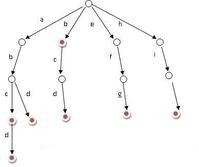它是一种哈希树的变种。典型应用是用于统计，排序和保存大量的字符串（但不仅限于字符串），所以经常被搜索引擎系统用于文本词频统计。它的优点是：利用字符串的公共前缀来节约存储空间，最大限度地减少无谓的字符串比较，查询效率比哈希表高。

输入的一组单词，创建Trie树。输入字符串，计算以该字符串为公共前缀的单词数。

（提示：树结点有26个指针，指向单词的下一字母结点。）

输入

测试数据有多组

每组测试数据格式为：

第一行：一行单词，单词全小写字母，且单词不会重复，单词的长度不超过10

第二行：测试公共前缀字符串数量t

后跟t行，每行一个字符串

输出

每组测试数据输出格式为：

第一行：创建的Trie树的层次遍历结果

第2~t+1行：对每行字符串，输出树中以该字符串为公共前缀的单词数。

输入样例

abcd abd bcd efg hig
3
ab
bc
abcde

输出样例

abehbcficddggd
2
1
0

参考代码

#include <iostream>
#include <stdio.h>
#include <vector>
#include <queue>
using namespace std;
struct Node{
char date;
struct Node *next;    //next指针有26个，代表26个字母
};
class Tire{
private:
struct Node *m;
public:
Tire(){};
~Tire(){};
void test();
int get_num(struct Node *t);
void level_print(struct Node *t);
};
void Tire::level_print(struct Node *t)    //层序遍历输出结果,t默认取树根
{
queue<struct Node*> q1;            //设置一个队列，先将根结点不空的孩子加进来
for(int i = 0; i < 26; i++)
{
if(t[i].date != '0')
{
q1.push(&t[i]);
}
}
while(!q1.empty())            //在队列不空的情况下，每次输出结点对应的字符，然后将结点的孩子中不空的结点加到队列中
{
struct Node *t = q1.front();
q1.pop();
cout<<t->date;
for(int i = 0; i < 26; i++)
{
if(t->next[i] != NULL)
{
q1.push(t->next[i]);
}
}
}
cout<<endl;
}
int Tire::get_num(struct Node *t)    //获取该结点的子树的个数，需要递归实现
{
int coun=0;
for(int i=0;i<26;i++)
{
if(t->next[i] != NULL)          //如果孩子结点不空，则计算此孩子结点的子树个数，加到父结点的子树个数中去
{
coun += get_num(t->next[i]);
}
}
if(coun == 0)        //若最后的计算结果显示所有孩子结点都是空，证明只含有父结点这棵树，长度就是1
return 1;
else
return coun;
}
void Tire::test()
{
m = new Node;
for(int i=0; i<26; i++)        //初始化结构体
{
m[i].date='0';
for(int j=0;j<26;j++)
m[i].next[j]=NULL;
}
char str;
int num=0;
while((str[num] = getchar()) != '\n')    //读入第一行输入的所有单词
num++;
for(int i=0;i<num;i++)
{
string t;                    //对单词进行分割，每次分割出来的单词存在t中
while(str[i] != ' ' && i<num)
{
t+=str[i];
i++;
}
struct Node *father = &m[t-'a'];        //设置父结点指针，方便单词加到字典树中
father->date = t;
for(int j = 1; j < t.length(); j++)
{
if(father->next[t[j]-'a'] != NULL)    //如果单词的第i个字母已经存在字典树中，则父节点继续往下，如果不存在，则生成新的结点加入到字典树中去
{
father=father->next[t[j]-'a'];
continue;
}
struct Node* temp = new Node;
temp -> date = t[j];
for(int k = 0; k < 26; k++)
temp->next[k] = NULL;
father->next[t[j]-'a'] = temp;
father = father->next[t[j]-'a'];
}
}
level_print(m);        //层序遍历字典树输出结果
int n;                //输入要检测的前缀的个数
cin>>n;
string temp;
for(int i=0;i<n;i++)
{
cin>>temp;        //输入前缀
struct Node *father = &m[temp-'a'];
for(int j=1;j<temp.length();j++)            //father指针取前缀的最后一个字母在树中的位置，如果不存在，则father指针为空
{
father = father->next[temp[j]-'a'];
if(father == NULL)
break;
}
if(father==NULL)            //若father指针为空，则前缀不存在字典树中，输出0
cout<<0<<endl;
else                        //若father指针不为空，则调用计算子树个树函数，输出father指针的子树的个数，此个数即为具有此公共前缀的单词的个数
{
int coun = get_num(father);
cout<<coun<<endl;
}
}

}
int main()
{
Tire t;
t.test();
return 0;
}

展开全文数据结构
• 2）熟练掌握哈希表的构建和哈希查找。 二、实验环境 1）自备计算机，windows操作系统以及相关的编译器（如Devc++）。 三、实验要求 1）能够分析并设计一个哈希表。哈希函数用除留余数法构造，用线性探测再散列处理...

一、实验目的

1）理解并熟练掌握哈希表和哈希函数，掌握各种哈希函数和冲突解决办法。
2）熟练掌握哈希表的构建和哈希查找。

二、实验环境

1）自备计算机，windows操作系统以及相关的编译器（如Devc++）。

三、实验要求

1）能够分析并设计一个哈希表。哈希函数用除留余数法构造，用线性探测再散列处理冲突。
2）用哈希表构建一份学生成绩报告单，满足任给任一学生学号即可打印出该生的成绩报告单。

四、实验内容

代码如下：

#include<stdio.h>
#include <time.h>
#include<conio.h> /*清屏*/
#include <stdlib.h> /*显示目录*/
#include<string.h>
#define MAX 666
void input(); /*输入数据函数*/
void sort();/*排序数据函数*/
void sort1();
void sort2();
void sort3();
void display();/*显示数据函数*/
void display1();
void insert(); /*插入数据函数*/
void del(); /*删除数据函数*/
void average(); /*平均值函数*/
void find();/*查找数据函数*/
void find1();
void find2();
void save(); /*保存数据函数*/
void del_file();  /*删除文件函数*/
void modify(); /*修改文件函数*/
int now_no=0;
struct student
{
int no;
char name;
char sex;
float score1;
float score2;
float score3;
float sort;
float ave;
float sum;
};
struct student stu[MAX],*p;
main()/*主函数*/
{
int as;
char ch;
do{

start: printf("\n\n\n\t\t\t欢迎使用小白的学生成绩管理系统\n");
printf("\n\n\n\n\n\n\t\t******************按任意键继续********************");
ch=getch();
}
while(!ch);
system("cls");
/*一下为功能选择模块*/
do
{
printf("\n\t\t\t\t1.录入学员信息\n\t\t\t\t2.显示学员总成绩信息\n\t\t\t\t3.对总成绩排序\n\t\t\t\t4.显示学员单科成绩排序\n\t\t\t\t5.添加学员信息\n\t\t\t\t6.删除学员信息\n\t\t\t\t7.修改学员信息\n\t\t\t\t8.查询学员信息\n\t\t\t\t9.从文件读入学员信息\n\t\t\t\t10.删除文件中学员信息\n\t\t\t\t11.保存学员信息\n\t\t\t\t12.退出\n");
printf("\t\t\t\t选择功能选项(输入所选功能前的数字):");
fflush(stdin);
/*可用可不用，用于清除缓存防止下次用scanf输入是出现错误*/
scanf("%d",&as);
switch(as)
{
case 1:system("cls");
input();
break;
case 2:system("cls");
display();
break;
case 3:system("cls");
sort();
break;
case 4:system("cls");
display1();
break;
case 5:system("cls");
insert();
break;
case 6:system("cls");
del();
break;
case 7:system("cls");
modify();
break;
case 8:system("cls");
find();
break;
case 9:system("cls");
break;
case 10:system("cls");
del_file();
break;
case 11:system("cls");
save();
break;
case 12:system("exit");
exit(0);
default:system("cls");
goto start;
}
}while(1);/*while(1)，1表示真，所以while(1)表示永远循环下去，一般在while(1)的循环体内都有break 或者return 跳出循环*/
/*至此功能选择结束*/
}

void input()/*原始数据录入模块*/
{
int i=0;
char ch;
do
{
printf("\t\t\t\t1.录入学员信息\n输入第%d个学员的信息\n",i+1);
printf("\n输入学生编号:");
scanf("%d",&stu[i].no);
fflush(stdin);
printf("\n输入学员姓名:");
fflush(stdin);
gets(stu[i].name);
printf("\n输入学员性别:");
fflush(stdin);
gets(stu[i].sex);
printf("\n输入学员成绩1:");
scanf("%f",&stu[i].score1);
printf("\n输入学员成绩2:");
fflush(stdin);
scanf("%f",&stu[i].score2);
printf("\n输入学员成绩3:");
fflush(stdin);
scanf("%f",&stu[i].score3);
printf("\n\n");
i++;
now_no=i;
printf("是否继续输入?(Y/N)");
fflush(stdin);
ch=getch();
system("cls");
}
while(ch!='n'&&ch!='N');
system("cls");
}
void sort()/*排序数据函数*/
{
struct student temp;
int i,j;
average();
for(i=1;i<now_no;i++)
{
for(j=1;j<=now_no-i;j++)
{
if(stu[j-1].ave<stu[j].ave)
{
temp=stu[j];
stu[j]=stu[j-1];
stu[j-1]=temp;
}
}
}
printf("排序以完成进入功能2可进行显示\n");
system("pause");
system("cls");
}
void sort1()/*排序数据函数*/
{
struct student temp;
int i,j;
for(i=1;i<now_no;i++)
{
for(j=1;j<=now_no-i;j++)
{
if(stu[j-1].score1<stu[j].score1)
{
temp=stu[j];
stu[j]=stu[j-1];
stu[j-1]=temp;
}
}
}
}
void sort2()/*排序数据函数*/
{
struct student temp;
int i,j;
for(i=1;i<now_no;i++)
{
for(j=1;j<=now_no-i;j++)
{
if(stu[j-1].score2<stu[j].score2)
{
temp=stu[j];
stu[j]=stu[j-1];
stu[j-1]=temp;
}
}
}
}
void sort3()/*排序数据函数*/
{
struct student temp;
int i,j;
for(i=1;i<now_no;i++)
{
for(j=1;j<=now_no-i;j++)
{
if(stu[j-1].score3<stu[j].score3)
{
temp=stu[j];
stu[j]=stu[j-1];
stu[j-1]=temp;
}
}
}
}
void display()/*显示数据函数*/
{
int i;
char as;
average();
do
{
printf("\t\t\t班级学员信息列表\n");
printf("\t编号\t姓名\t性别\t成绩1\t成绩2\t成绩3\t平均值\n");
for(i=0;i<now_no&&stu[i].name;i++)
printf("\t%d\t%s\t%s\t%.2f\t%.2f\t%.2f\t%.2f\n",stu[i].no,stu[i].name,stu[i].sex,stu[i].score1,stu[i].score2,stu[i].score3,stu[i].ave);
printf("\t\t按任意键返回主菜单.");
fflush(stdin);
as=getch();
}
while(!as);
system("cls");
}
void display1()/*显示数据函数*/
{
int i;
char as;
do
{
printf("\t\t\t班级学员score1成绩排序\n");
printf("\t编号\t姓名\t性别\t成绩1\n");
sort1();
for(i=0;i<now_no&&stu[i].name;i++)
printf("\t%d\t%s\t%s\t%.2f\t\n",stu[i].no,stu[i].name,stu[i].sex,stu[i].score1);
printf("\t\t\t班级学员score2成绩排序\n");
printf("\t编号\t姓名\t性别\t成绩2\n");
sort2();
for(i=0;i<now_no&&stu[i].name;i++)
printf("\t%d\t%s\t%s\t%.2f\t\n",stu[i].no,stu[i].name,stu[i].sex,stu[i].score2);
printf("\t\t\t班级学员score3成绩排序\n");
printf("\t编号\t姓名\t性别\t成绩3\n");
sort3();
for(i=0;i<now_no&&stu[i].name;i++)
printf("\t%d\t%s\t%s\t%.2f\t\n",stu[i].no,stu[i].name,stu[i].sex,stu[i].score3);
printf("\t\t按任意键返回主菜单.");
fflush(stdin);
as=getch();
}
while(!as);
system("cls");
}

void insert()/*插入数据函数*/
{
char ch;
do
{
printf("\n\t\t输入新插入学员队信息\n");
printf("\n输入学生编号:");
scanf("%d",&stu[now_no].no);
fflush(stdin);
printf("\n输入学员姓名:");
fflush(stdin);
gets(stu[now_no].name);
printf("\n输入学员性别:");
fflush(stdin);
gets(stu[now_no].sex);
printf("\n输入学员成绩1:");
fflush(stdin);
scanf("%f",&stu[now_no].score1);
printf("\n输入学员成绩2:");
fflush(stdin);
scanf("%f",&stu[now_no].score2);
printf("\n输入学员成绩3:");
fflush(stdin);
scanf("%f",&stu[now_no].score3);
printf("\n\n");
now_no=now_no+1;
sort();
printf("是否继续输入?(Y/N)");
fflush(stdin);
ch=getch();
system("cls");
}
while(ch!='n'&&ch!='N');
}
void del()/*删除数据函数*/
{
int inum,i;
printf("输入要删除学员的编号:");
fflush(stdin);
scanf("%d",&inum);
for(i=0;i<now_no;i++)
{
if(stu[i].no==inum)
{
if(i==now_no)now_no-=1;
else
{
stu[i]=stu[now_no-1];
now_no-=1;
}
sort();
break;
}
}
system("cls");
}
void save()/*保存数据函数*/
{
FILE *fp;
int i;
char filepath;
printf("输入要保存的文件路径:");
fflush(stdin);
gets(filepath);
if((fp=fopen(filepath,"w"))==NULL)
{
printf("\n保存失败!");
exit(0);
}
for(i=0;i<now_no;i++)
{
stu[i].sum=stu[i].score1+stu[i].score2+stu[i].score3;
stu[i].ave=stu[i].sum/3;
fprintf(fp,"\t%d\t%s\t%s\t%.2f\t%.2f\t%.2f\t%.2f\n",stu[i].no,stu[i].name,stu[i].sex,stu[i].score1,stu[i].score2,stu[i].score3,stu[i].ave);
}
fclose(fp);
printf("学生信息已保存在%s中!\n",filepath);
system("pause");
system("cls");
}
void find()/*查询函数*/
{
int i;
char str,as;
do
{
printf("输入要查询的学生姓名:");
fflush(stdin);
gets(str);
for(i=0;i<now_no;i++)
if(!strcmp(stu[i].name,str))
{
printf("\t编号\t姓名\t性别\t成绩1\t成绩2\t成绩3\t平均值\n");
printf("\t%d\t%s\t%s\t%.2f\t%.2f\t%.2f\t%.2f\n",stu[i].no,stu[i].name,stu[i].sex,stu[i].score1,stu[i].score2,stu[i].score3,stu[i].ave);
}
printf("\t\t按任意键返回主菜单.");
fflush(stdin);
as=getch();
}
while(!as);
system("cls");
}

void average()/*求平均数*/
{
int i;
for(i=0;i<now_no;i++)
{
stu[i].sum=stu[i].score1+stu[i].score2+stu[i].score3;
stu[i].ave=stu[i].sum/3;
}
}
void modify()/*修改数据函数*/
{
int i;
char str;
printf("输入要修改的学生姓名:");
fflush(stdin);
gets(str);
for(i=0;i<now_no;i++)
{
if(!strcmp(stu[i].name,str))
{
system("cls");
printf("\n\t\t输入新插入学员队信息\n");
printf("\n输入学生编号:");
fflush(stdin);
scanf("%d",&stu[i].no);
printf("\n输入学员性别:");
fflush(stdin);
gets(stu[i].sex);
printf("\n输入学员成绩1:");
fflush(stdin);
scanf("%f",&stu[i].score1);
printf("\n输入学员成绩2:");
fflush(stdin);
scanf("%f",&stu[i].score2);
printf("\n输入学员成绩3:");
fflush(stdin);
scanf("%f",&stu[i].score3);
printf("\n\n");
sort();
break;
}
}
system("cls");
}

{
FILE *fp;
int i;
char filepath;
printf("输入要读入的文件路径:");
fflush(stdin);
gets(filepath);
if((fp=fopen(filepath,"r"))==NULL)
{
printf("找不到%s文件!\n",filepath);
system("pause");
exit(0);
}
now_no=0;
for(i=0;i<MAX&&!feof(fp);i++)
{
fscanf(fp,"\t%d\t%s\t%s\t%f\t%f\t%f\t%f\n",&stu[i].no,stu[i].name,stu[i].sex,&stu[i].score1,&stu[i].score2,&stu[i].score3,&stu[i].ave);
now_no++;
}
fclose(fp);
printf("保存的在文件%s中的所有信息已经读入!\n",filepath);
system("pause"); /*按任意键继续*/
system("cls");
}

void del_file()
{
FILE *fp;
char filepath;
printf("输入要删除的文件路径:");
fflush(stdin);
gets(filepath);
fp=fopen(filepath,"w");
fclose(fp);
printf("保存的在文件%s中的所有信息已经删除!\n",filepath);
system("pause");
system("cls");
}

五、运行结果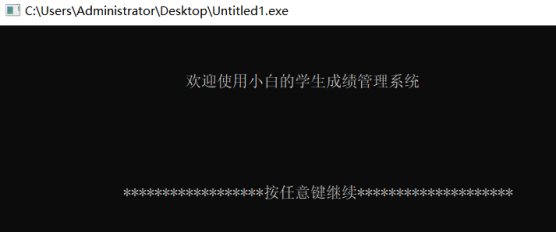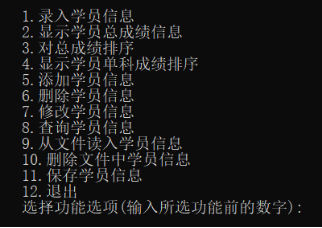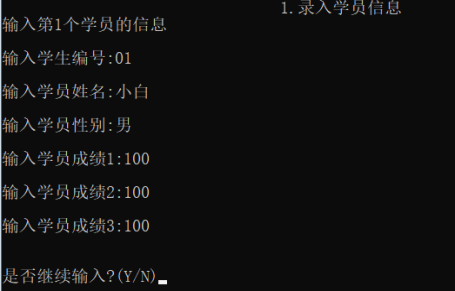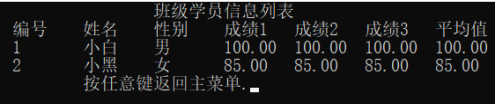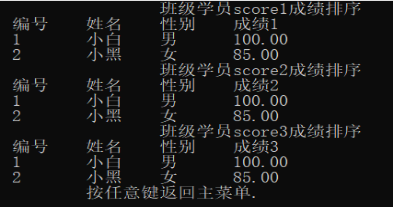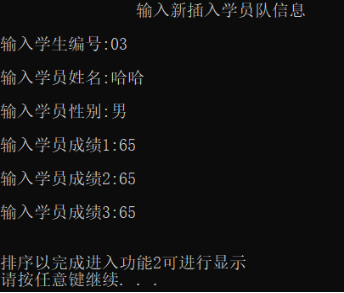六、实验总结

通过这次实验，我理解并熟练掌握了哈希表和哈希函数，能够使用各种哈希函数和冲突解决办法，熟练掌握哈希表的构建和哈希查找，更加深入地了解了散列函数。能够通过进一步的学习，分析并设计一个哈希表，用除留余数法构造哈希函数，用线性探测散列处理冲突，构建一个简单的学生成绩管理系统，实现多样化的操作。

展开全文算法 数据结构 哈希算法 c++
• /*8622 哈希查找 时间限制:1000MS 代码长度限制:10KB 提交次数:2013 通过次数:1250 题型: 编程题 语言: G++;GCC Description 使用哈希函数：H(k)=3*k MOD length，并采用开放定址法处理冲突。试对输入的关键字序列...
/*8622 哈希查找
时间限制:1000MS  代码长度限制:10KB
提交次数:2013 通过次数:1250

题型: 编程题   语言: G++;GCC
Description 使用哈希函数：H(k)=3*k MOD length，并采用开放定址法处理冲突。试对输入的关键字序列构造哈希表，哈希表长度为length，
求等概率情况下查找成功的平均查找长度，并设计构造哈希表的完整的算法。本题给出部分代码，请补全Hash函数和解决冲突的collison函数。*/
#include"malloc.h" /* malloc()等 */
#include"stdlib.h" /* exit() */
#include"stdio.h"
#define EQ(a,b) ((a)==(b))   //EQEQ(a,b) 等效于 ((a)==(b))
#define SUCCESS 1
#define UNSUCCESS 0
#define NULLKEY -1 /*哈希表无元素时值为-1*/
typedef int ElemType;
int length;
typedef struct
{
ElemType *elem; /* 数据元素存储基址，动态分配数组 */
int count; /* 当前数据元素个数 */
} HashTable;

void InitHashTable(HashTable *H)
{
/* 操作结果: 构造一个长度为length的哈希表,length为全局变量 */
int i;
(*H).count = 0; /* 当前元素个数为0 */
(*H).elem = (ElemType*)malloc(length * sizeof(ElemType));
if (!(*H).elem)
exit(0); /* 存储分配失败 */
for (i = 0; i < length; i++)
(*H).elem[i] = NULLKEY; /* 未填记录的标志 */
}
unsigned Hash(ElemType K)  //unsigned 等效于unsigned int,
{
/* 一个简单的哈希函数*/

return 3 * K % length;  //这里填空

}
void collision(int *p) /*线性探测再散列 */
{
/* 开放定址法处理冲突 */

*p = (*p + 1) % length; //这里填空  +1其实偏移量

}
int SearchHash(HashTable H, ElemType K, int *p, int *c)
{
/* 在开放定址哈希表H中查找关键码为K的元素,若查找成功,以p指示待查数据 */
/* 元素在表中位置,并返回SUCCESS;否则,以p指示插入位置,并返回UNSUCCESS */
/* c用以计冲突次数，其初值置零，供建表插入时参考。算法9.17 */
*p = Hash(K); /* 求得哈希地址 */
while (H.elem[*p] != NULLKEY && !EQ(K, H.elem[*p]))
{
/* 该位置中填有记录,并且关键字不相等 */
(*c)++;
if (*c < length)
collision(p); /* 求得下一探查地址p */
else
break;
}
if EQ(K, H.elem[*p])
return SUCCESS; /* 查找成功，p返回待查数据元素位置 */
else
return UNSUCCESS; /* 查找不成功(H.elem[p].key==NULLKEY)，p返回的是插入位置 */
}
int InsertHash(HashTable *H, ElemType e)
{
/* 查找不成功时插入数据元素e到开放定址哈希表H中，并返回查找长度 */
int c, p;
c = 0;
if (SearchHash(*H, e, &p, &c))   /* 表中已有与e有相同关键字的元素 */
printf("哈希表中已有元素%d。\n", e);
else  /* 插入e */
{
(*H).elem[p] = e;
++(*H).count;
}
return c + 1; /*查找长度为冲突次数加1*/
}
void TraverseHash(HashTable H)
{
/* 按哈希地址的顺序打印哈希表，无元素位置用X表示 */
int i;
for (i = 0; i < length; i++)
if (H.elem[i] == NULLKEY) /* 有数据 */
printf("X ");
else
printf("%d ", H.elem[i]);
printf("\n");
}
int main()
{
float i = 0, j = 0;
ElemType e;
HashTable H;
//printf("Input Table length:");
scanf("%d", &length);
InitHashTable(&H);
//printf("Input key words sequence, -1 conclusion input：");
scanf("%d", &e);
while (e != -1)
{
j++;  /*j记录输入元素个数*/
i = i + InsertHash(&H, e);  /*i记录查找长度的和*/
scanf("%d", &e);
}
TraverseHash(H);
printf("Average search length=%f\n", i / j);
}

/*
输入格式
第一行：输入哈希表的长度；
第二行：输入关键字序列，用空格分隔，-1结束(-1不作为关键字)。

输出格式
第一行：输出哈希表里的数据，未使用的单元用X表示；
第二行：输出平均查找长度,格式为"Average search length="。

输入样例
11
22 41 53 46 30 13 1 67 -1

输出样例
22 X 41 30 1 53 46 13 67 X X
Average search length=2.000000*/

展开全文• 顺序查找 二分查找 索引查找 哈希查找数据结构与算法】数据结构 二分查找 哈希算法 顺序查找 折半查找
• 哈希函数 有一种函数，根据这个函数和查找关键字key，...哈希表（Hash table，也叫散列表），是根据关键码值(Key value)而直接进行访问的数据结构。也就是说，它通过把关键码值映射到表中一个位置来访问记录，以加快数据结构 哈希表 算法
• 通过本实验的学习，理解哈希表的构造原理及构造方法，进一步理解通过哈希表的使用提高查找效率的原理，为不同数据结构选择合适的查找算法奠定基础。 二、实验内容 【问题描述】 针对你所在的班级中的“人名”设计一...数据结构 哈希表 c语言
• 数据结构 实验十二：（查找实验，设计性） 代码 /* 时间：2021/06/20 作者：贾瑞龙 班级：191104 学号：201934110424 功能：通过姓名 建立哈希表 */ #include <stdio.h> #include <string.h> #...数据结构 哈希表 c语言
• 整理内容来源：zzu信息工程学院数据结构ppt 本节讨论两类不同的查找表：静态查找表和动态查找表，给出在不同查找表上进行查找的不同算法和性能分析以及动态查找表的创建方法。 1 查找的基本概念 静态查找： 基于...数据结构 算法 数据库 查找算法
• 哈希查找算法

2021-10-11 10:21:16
哈希查找算法又称散列查找算法，是一种借助哈希表（散列表）查找目标元素的方法，查找效率最高时对应的时间复杂度为 O(1)。 哈希查找算法适用于大多数场景，既支持在有序序列中查找目标元素，也支持在无序序列中查找...算法 数据结构 线性代数
• 给定一组查找关键字(32,15,7,11,4,28,56,61,79)，哈希表长为m=12，请按照除留余数法设计一个哈希函数，设每个记录的查找概率相等。 （1）画出按照线性探测再散列处理冲突得到的哈希表（给出求解过程），并计算等...数据结构
• 哈希查找算法的思想：通过对元素的关键字值进行某种运算，直接求出元素的地址，即使用关键字到地址的直接转换方法，而不需要反复比较。 直接转换方法有很多，这里介绍最常用的一种方法：除留取余法，即H（key）=key...数据结构 哈希算法 c语言
• 狭义：数据结构具备的功能。 算法的特征： 有穷性、确切性、输入项、输出项、可行性 算法的评定： 正确性、时间复杂度、空间复杂度、可读性、健壮性 时间复杂度： 一个用于描述算法在执行时，随着输入参数数量的变化...算法 数据结构 c语言 二分查找 哈希算法
• 数据结构作业——顺序、二分、哈希查找代码实现 代码实现 #pragma GCC optimize ("O3") #include<iostream> #include<cstdio> #include<cstring> #include<cmath> #include<string> #...数据结构 哈希
• 散列表是根据关键字直接进行访问的数据结构。散列表通过散列函数将关键字映射到存储地址，建立了关键字和存储地址之间的一种直接映射关系。这里的存储地址可以是数组下标、索引、内存地址等。 利用哈希查找元素...数据结构 算法 散列表 哈希算法
• 哈希表是一种数据结构，通过哈希函数来组织数据，以支持快速插入和搜索。 哈希表的关键思想是使用哈希函数将键映射到存储桶。更确切地说， 当我们插入一个新的键时，哈希函数将决定该键应该分配到哪个桶中，并将该...数据结构 散列表 哈希算法
• 哈希表（Hash Table，也叫散列表），是根据键（Key）而直接访问在内存存储位置的数据结构。 也就是说，它通过计算一个关于键值的函数，将所需查询的数据映射到表中一个位置来访问记录，这加快了查找速度。这个映射...
• 哈希查找

千次阅读 2021-02-06 19:57:51
如果对一批数据要进行反复的搜索，用哈希查找是很好的。 在查找以前先建哈希表(分组)：哈希散列函数，常用方法为求整取余(p=key%m，把m定成n值)，然后入表，把对应的值放在正确的位置上，但是假如说数据中有1个数字...算法 数据结构
• 本节我们走得更远一些，创建一个数据结构，使得查找性能提高到O(1)。称为哈希查找。要做到这种性能，我们要知道元素的可能位置。假设每一个元素就在他应该在的位置上，那么要查找的时候仅仅须要一次比較得到有没有的...
• 哈希查找实现

2021-12-12 12:07:50
第七章查找数据结构作业 date:2021/12/10 content:哈希查找实现(线性探测法处理冲突) mind: 1.创建哈希表 2.核心代码是线性探测，向右顺位思想，走到尾无位置，再从头开始顺位 测试用例为课本232页第5题 散列表的...哈希算法 散列表 数据结构
• 数据结构实验报告五 查找

千次阅读 2020-12-31 13:45:44数据结构
• 哈希表概念 决定一个哈希表的主要是哈希函数与处理冲突的方法。而按照设定的哈希函数和处理冲突的方法将一组关键字key 映射到有限的地址集合中，这就是哈希表。 哈希函数构造方法 直接定义法：代码块如下： int ...数据结构 c语言
• （4）从散列文件中查找一个元素。 散列文件通常采用链接法处理冲突。 散列文件中每个节点的类型定义为： Struct FLNode { //散列主文件中的节点类型 ElemType data ; //值域 Int next; //指向下一个节点的指针域 }; ...数据结构 c语言 哈希表
• 哈希表(Hash Table)也叫散列表，是根据关键码值（Key Value）而直接进行访问的数据结构。它通过把关键码值映射到哈希表中的一个位置来访问记录，以加快查找的速度。这个映射函数就做散列函数，存放记录的数组叫做散...数据结构 哈希表
•数据结构 散列表 哈希
• 第一次结束哈希表是在数据结构课上，在讲查找的时候老师随便提了一下哈希表这个概念，最近在做聊天室的时候要用到哈希表，更加深入的理解了哈希表。 1.什么是HashMap? 先说说存储结构，实际上在我们学过的数据结构...数据结构 链表
• MySQL哈希索引的数据结构以及索引的优缺点

千次阅读 多人点赞 2021-08-03 11:27:39
MySQL哈希索引结构的实现，以及索引的优缺点。mysql mysql索引 哈希索引 索引的优缺点
• 哈希查找”算法是一个优秀的算法，在理想情况下，时间复杂度为O(1)，当然了，哈希查找非常依赖哈希函数，劣质的哈希函数有可能让哈希查找的时间复杂度退化为O(n)。文章的最后，使用了C++中的STL映射map解决了...c语言哈希查找算法
• 哈希表设计：针对某个集体中人名设计一个哈希表，使得平均查找长度不超过2，并完成相应的建表和查表程序。数据结构 哈希表
• Trie树又称单词查找树，是一种树形结构，如下图所示。 它是一种哈希树的变种。典型应用是用于统计，排序和保存大量的字符串（但不仅限于字符串），所以经常被搜索引擎系统用于文本词频统计。它的优点是：利用字符...哈希算法 散列表 数据结构...

哈希查找数据结构报告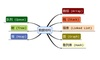数据结构 订阅